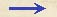Discorsi Propositions 3/08-th-05 Discorsi Proposition3/08-th-05{297} THEOREMA, PROPOSITIO VIII. {297} THEOREM. PROPOSITION VIIIAmplitudines parabolarum a proiectis eodem impetu explosis factarum, iuxta elevationes per angulos aequales supra et infra a semirecto distantes, aequales sunt inter se. The amplitudes of two parabolas described by projectiles (Condition Oblique-project-sym) fired with the same speed, but at angles of elevation which exceed and fall short of 45° by equal amounts, are equal to each other. Trianguli mcb circa angulum rectum c sint horizontalis bc et perpendicularis cm aequales; sic enim angulus mbc semirectus erit: et extensa cm in d, supra et infra diagonalem mb constituantur in b duo anguli aequales, mbe, mbd: demonstrandum est, amplitudines parabolarum a proiectis explosis {10} eodem impetu ex termino b iuxta elevationes angulorum ebc, dbc, esse aequales.Quia enim angulus externus bmc internis mdb, dbm est aequales, iisdem aequabitur quoque angulus mbc: quod si loco anguli dbm ponamus mbe, erit idem angulus mbc duobus mbe, bdc aequalis; et dempto communi mbe, reliquus bdc reliquo ebc erit aequalis: sunt igitur trianguli dcb, bce similes.Dividantur rectae dc, ec bifariam in h et f, et ducantur hi, fg horizontali cb aequidistantes, et ut dh ad hi, ita fiat ih ad hl: erit triangulus ihl similis triangulo ihd, cui etiam {20} similis est egf; cumque ih, gf sint aequalis (dimidiae nempe ipsius bc), erit fe, id est fc, aequalis hl; et addita communi fh, erit ch ipsi fl aequalis.Si itaque intelligamus, per h et b semiparabolam esse descriptam, cuius altitudo erit hc, sublimitas vero hl, erit amplitudo eius cb, quae dupla est ad hi, media scilicet inter dh, seu ch, et hl; eamque tanget db, aequalibus existentibus ch, hd.Quod si, rursus, parabolam per fb descriptam concipiamus a suplimitate fl cum altitudine fc, quarum media proportionalis est fg, {298} cuius dupla est horizontalis cb, er pariter cb eius amplitudo, illamque tanget eb, cum ef, fc sint aequales: distant autem anguli dbc, ebc (elevationes scilicet ipsarum) aequaliter a semirecto patet propositum. In the triangle mcb let the horizontal side bc and the vertical cm, which form a right angle at c, be equal to each other; then the angle mbc will be a semi-right angle; let the line cm be prolonged to d, such a point that the two angles at b, namely mbe and mbd, one above and the other below the diagonal mb, shall be equal. It is now to be proved that in the case of two parabolas described by two projectiles fired from b with the same speed, one at the angle of ebc, the other at the angle of dbc, their amplitudes will be equal. Now since the external angle bmc is equal to the sum of the internal angles mdb and dbm we may also equate to them the angle mbc; but if we replace the angle dbm by mbe, then this same angle mbc is equal to the two mbe and bdc: and if we subtract from each side of this equation the angle mbe, we have the remainder bdc to the remainder ebc. Hence the two triangles dcb and bce are similar. Bisect the straight lines dc and ec in the points h and f: and draw the lines hi and fg parallel to the horizontal cb, and choose l such that dh:hi = ih:hl. Than the triangle ihl will be similar to ihd, and also the triangle egf; and since ih and gf are equal being half of bc, it follows that hl is equal to fe and also to fc; and if we add to each of these the common part fh, it will be seen that ch is equal to fl. Let us now imagine a parabola described through the points h and b whose altitude is hc and sublimity hl. Its amplitude will be cb which is double the length hi (Condition 3/05-pr-02-cor) since hi is a mean proportional between dh (or ch) and hl. The line db is tangent to the parabola at b, since ch is equal to hd. (Condition 3/05-pr-02-cor) If again we imagine a parabola described through the points f and b, with a sublimity fl and altitude fc, of which the mean proportional is fg, or one-half of cb, then, as before, will cb be the amplitude and the line eb a tangent at b; for ef and fc are equal. {298} But the two angles dbc and ebc, the angles of elevation, differ by equal amounts from a 45° angle. (Condition 3/10-th-07) Hence follows the proposition.Discorsi Propositions 3/08-th-05 Discorsi Proposition3/08-th-05